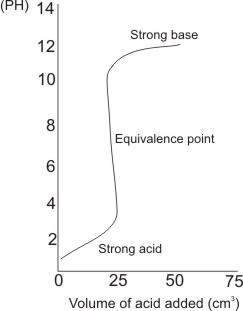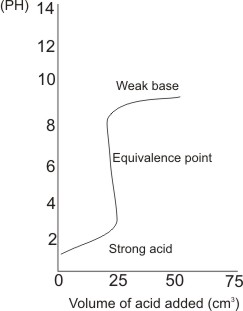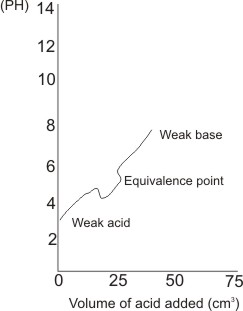## Acid-Base Indicators

An acid/base indicator is a chemical compound used to indicate the pH values of substances by giving a recognizable color change. Hence, it can be used to detect the equivalent point (or end-point) during an acid-base titration process. Equivalent point is the point at which equivalent volume of the acid have reacted with the alkali. There are two types of acid/base indicators:

1. Universal indicator - this is a mixture of several indicators. It can indicate pH values of over a range of 3 -11 by successive changes of color - this takes place at every change of one unit of pH.

2. Ordinary single indicator – this requires a change of about two units of pH to show a full color change. The table below gives the three common indicators for acid - alkali titration.

 Indicator Acid Side Neutral Alkaline Side Methyl Orange Pink Orange Yellow Litmus Red Purple Blue Phenolphthalein Colorless Colorless Pink

### Choice of Indicator

lt is possible to calculate before hand a rough value of the pH of the mixture at end point of an acid/base titration, hence any one of the indicators which give color change at or close to the calculated pH value is suitable to be used for the titration. Therefore, the choice of an indicator in an acid/base titration actually depends on the strength of acids and bases involved and how well the colors can be distinguished by the eyes.

Below are a number of typical acid/base titrations (0.1 M of 25 cm3 of base is titrated upon by 0.1 M of the acid, which is added in small quantities). For a strong acid and a strong base titration (e.g. HCl and NaOH) Methyl orange is a suitable indicator for this titration.

Methyl orange changes color over a pH range of 3.2 - 4.4. At pH of 4.4, methyl orange gives a full color change, indicating the equivalence point. Methyl orange gives color change very close to the equivalence point, with an error of less than 0.1%. Methyl red (pH range of 4.8 - 6.0) is also suitable for strong acid and strong base titrations.

Graphically:Note: - There is a sharp drop in pH near or at the equivalence point. - The titre value, i.e., the volume of acid added at equivalence point is deduced by tracing the almost vertical straight line in the graph to the horizontal axis. For the titration whose graph is plotted, the titre value is 25 cm3.

- The pH of the solution at the start (when no acid was added) is beyond 12. This indicates strong base (i.e. the base used is a strong base). At the end of the process (when the concentration of the solution becomes slightly acidic), the pH is close to 1, indicating the use of a strong acid.

For a weak acid and a strong base (e.g acetic or ethanoic acid and NaOH) A suitable indicator is phenolphthalein. The pH of the mixture at the equivalence point is 8.73 – phenolphthalein gives color change between the pH range of 8.2 to 10.0.

Graphically:Note: the pH of the solution when no acid was added is beyond 12, indicating a strong base, while the pH slightly beyond the equivalence point is close to 3 - indicating weak acid.

The titre value is 25 cm3.

There is a sharp drop in pH near or at equivalence point. For a strong acid and a weak base (e.g. HCl and NH3) the pH of the mixture of strong acid and week base at equivalence point is 5.28, therefore, indicators which change color at or close to this point is suitably used. The same kind of indicators for strong acid and strong base titrations can be used - i.e., methyl orange and methyl red.

Graphically:For a weak acid and a weak base Color indicators are not suitable for use to indicate the end-point (equivalence point). Color indicators are useful if small quantities of either titrant is needed to produce sharp recognizable color change. However, for weak acid/base titration, large quantities of titrants are needed if color change is to from - this results in huge errors.

Graphically:Note: there is no sharp drop in pH near or at the equivalent point. Titration of weak polyprotic acids Polyprotic acids are acids which have more than one ionizable hydrogen. E.g. H2CO3 and H3PO4. The hydrogens are ionized one at a time. Their titration curves show equivalence points corresponding to the number of ionizable hydrogen atoms contained.

Graph of titration of a strong base with diprotic acid, e.g. H2CO3.Like This Post? Please Share!!

Copyright , All Rights Reserved Free Chemistry Online | About Us | Usage of Content | Total Disclosures | Privacy Policy